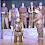## 27 January 2014

### What is the distance between these poles?

Two poles stand vertically on level ground. One is 10 feet tall, the other 15 feet tall. If a line is drawn from the top of each pole to the bottom of the other, the two lines intersect at a point 6 feet above the ground. What’s the distance between the poles?
You can take a wild guess (and be correct), or view the answer at Futility Closet.

Addendum: If you're like me and need a bit of help in understanding the answer, see the comment by the fourth Anonymous (8:28 PM), which refers to this annotated version of the same diagram:

1.Can't tell. No matter what the spacing between the poles, the intersection of the top-to-bottom lines will always be 6 feet.

1.Can you explain why? True, 15x10/15+10 = 6, but how does that apply?

2.Stared at this for 10 minutes. Stared some more - math, obviously, wasn't my subject. Finally gave up and clicked the link,and what do I get? "502 Bad Gateway"

You're a cruel man, Stan.

1.The Futility Closet home page is also inaccessible tonight. Someone must have locked the door.

3.This comment has been removed by the author.

1.Are you figuring the tangents of equivalent angles? If so, I don't think that the angles you're equating are equal. I'd say:
4/x1 = 6/x2 = 10/x
9/x2 = 6/x1 = 15/x
which is also a way of showing the slopes of the two diagonals.

I think JPS above is right; the intersection of the lines, regardless of x1 + x2, will always be at 6 high, just as x1:x2 will always be 2:3. Try figuring x1 and x2 for total x of 10 and 100 separately and see what that gives you.

4.Those lines will always intersect at 6' up unless far enough apart for the curvature of the surface of the world (or simple topography) to matter

1.Why? Because... math, sure. But can you ELI5?

5.I can try, using (https://www.dropbox.com/s/womlsye6da89t02/pole_position.jpg) as a reference.

For starters, the slope of a line is figured as rise / run. So the slope of line L is 10/C, and the slope of line R is 15/C.

To get to X, we can see that the rise of line L over distance B = rise of line R over distance A.

The run of a line over a certain distance is the rise / slope, so for line L over distance B, it's X / (10/C). Since (X/1) / (10/C) can be said (X/1) * (C/10), we get to the run for line L over distance B, or simply distance B = XC/10.

Going through the same exercise with the distance A and slope of line R (15/C), we get that A = XC/15.

A + B = C, so by swapping out terms, we get XC/15 + XC/10 = C. To further simplify, let's make those denominators common. The least common multiple of 10 and 15 is 30, so by multiplying the first term by 2/2 and the second by 3/3, we get 2XC/30 + 3XC/30 = C. Add 'em up to get 5XC/30 = C.

Factor the left side (divide top and bottom by 5) to arrive at XC/6 = C. Looks like we can divide both sides by C to get X/6 = 1. We want to see the X stand alone, so multiply both sides by 6, finally getting X = 6.

Hope that helps!

1.Thank you! Now I can understand.

I've incorporated your dropbox diagram into the body of the post.

2.Also, if instead of using 30 as the denominator you use 150, you get

10XC/150 + 15XC/150 = C or (10 + 15)XC / (10*15)= C

Divide both sides by XC to get

(10+15) / (10*15) = 1/X

Now flip numerator and denominator to get the Futilityn Closet's equation:

(10*15)/(10+15) = 6

6.What others have said. Using similar triangles, it's easy enough to figure out the 3:2 ratio between B:A and 15:10 and the parts of L and R. Couldn't progress much from there, however, until I tried using Cartesian equations. Any line is definable by using y = mx + b, where m is the slope and b is the y-intercept. Using that, and setting the origin at the bottom left edge, we get

f(x) = 15/(A+B) + 0 and

g(x) = -10/(A+B) + 10

We also know that f(A) = 6 and g(A) = 6

From f(A) we get 6A + 6B = 15A and
from g(A) we get 4A + 4B = 10A

which gives us no new information. The implication is that we don't have enough information
in the problem. So I tried A = 2 and B = 3 (keeping the 3:2 ratio), and f(2) = g(2) = 6.
Then I tried A = 4 and B = 6, and still f(4) = g(4) = 6. The intersection point will always be 6 high.

Weird.

Lurker111